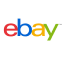New update is available. Click here to update.
Topics

# Strange Numbers

Hard0/120
Average time to solve is 45m## Problem statement

Alice is very fond of digits. While she was playing with digits from 0 to 9, she noticed that when digits 0, 1, 6, 8, 9 are rotated 180 degrees, they become 0, 1, 9, 8, 6, respectively, and when digits 2, 3, 4, 5, and 7 are rotated 180 degrees, they become invalid.

After noticing such a property of digits, she started considering some numbers as strange numbers. According to her, a strange number is a number that, when rotated 180 degrees in a clockwise fashion, becomes a different number with each digit valid. As she is busy playing with digits, she gave you an integer ‘N’ and asked you to find the number of strange numbers from 1 to ‘N’ inclusive.

Note :

``````The rotated number can be greater(or smaller) than the original number.
``````
Detailed explanation ( Input/output format, Notes, Images )

#### Sample Input 1 :

``````2
5
10
``````

#### Sample Output 1 :

``````0
3
``````

#### Explanation For Sample Input 1 :

``````Test case 1:
Numbers from 1 to 5 are 1, 2, 3, 4, 5.
1, when rotated 180 degrees, remains the same.
2, 3, 4, 5, when rotated 180 degrees, becomes invalid.
Therefore there are 0 strange numbers from 1 to 5.

Test case 2:
Strange numbers from 1 to 10 inclusive are 6, 9, and 10.
6, when rotated 180 degrees, becomes 9.
9, when rotated 180 degrees, becomes 6.
10, when rotated 180 degrees, becomes 01, which is the same as 1.
``````

#### Sample Input 2 :

``````1
16
``````

#### Sample Output 2:

``````4
``````

#### Explanation For Sample Input 2 :

``````Test case 1:
Strange numbers from 1 to 16 inclusive are 6, 9, 10, and 16.
6, when rotated 180 degrees, becomes 9.
9, when rotated 180 degrees, becomes 6.
10, when rotated 180 degrees, becomes 01, which is the same as 1.
16, when rotated 180 degrees, becomes 91.
``````Console